# Mechanical Engineering - Hydraulics and Fluid Mechanics

### Exercise :: Hydraulics and Fluid Mechanics - Section 5

41.

A differential manometer is used to measure

 A. atmospheric pressure B. pressure in pipes and channels C. pressure in venturimeter D. difference of pressures between two points in a pipe

Answer: Option D

Explanation:

No answer description available for this question. Let us discuss.

42.

The metacentric height of a ship is 0.6 m and the radius of gyration is 4 m. The time of rolling of a ship is

 A. 4.1 s B. 5.2 s C. 10.4 s D. 14.1 s

Answer: Option C

Explanation:

No answer description available for this question. Let us discuss.

43.

The point at which the resultant pressure on an immersed surface acts, is known as

 A. centre of gravity B. centre of depth C. centre of pressure D. centre of immersed surface

Answer: Option C

Explanation:

No answer description available for this question. Let us discuss.

44.

A tank of uniform cross-sectional area (A) containing liquid upto height (H1) has an orifice of cross-sectional area (a) at its bottom. The time required to bring the liquid level from H1 to H2 will be

 A.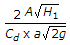B.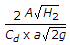C.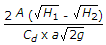D.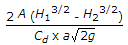Answer: Option C

Explanation:

No answer description available for this question. Let us discuss.

45.

The torque required to overcome viscous resistance of a collar bearing is (where R1 and R2 = External and internal radius of collar)

 A.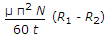B.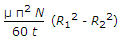C.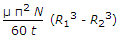D.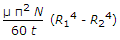Answer: Option D

Explanation:

No answer description available for this question. Let us discuss.

#### Current Affairs 2021

Interview Questions and Answers